# seaborn 入门

seaborn 是一个基于 matplotlib 的 Python 数据可视化库。它提供了一个高级界面，用于绘制有吸引力且信息丰富的统计图形。目前最新的版本是 v0.9.0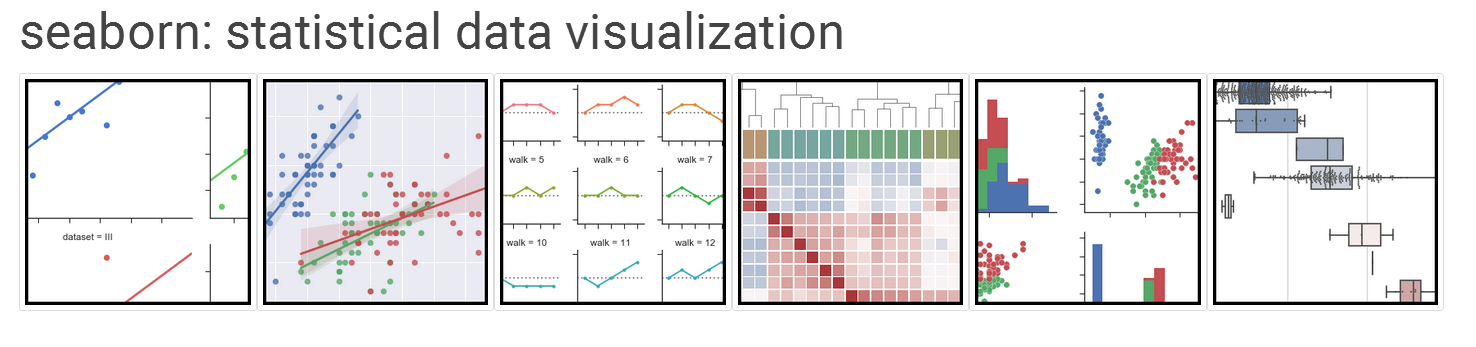## seaborn 安装

``````pip install seaborn
``````

## seaborn 使用

``````import seaborn as sns
import numpy as np
import matplotlib.pyplot as plt
import matplotlib as mpl

# 不需要调用show方法便可以直接显示当前的绘图
%matplotlib inline
``````

seaborn 是做为 matplotlib 的补充存在的，主要的画图操作还是由 matplotlib 来实现的，而 seaborn 主要是给画出的图像设置一些样式。

### 样式

seaborn 所提供的主题风格主要有五种：

• darkgrid
• whitegrid
• dark
• white
• ticks

``````def sinplot(flip=1):
# 创造100个随机数据
x = np.linspace(0, 14, 100)
# 画出六条图像
for i in range(1,7):
plt.plot(x, np.sin(x + i * .5) * (7 - i) * flip)
``````

``````sinplot()
``````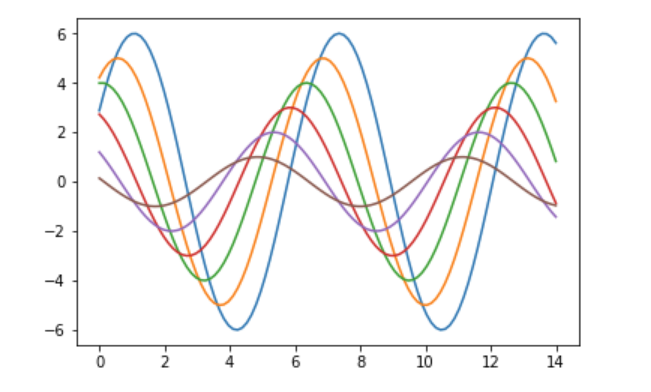``````sns.set()
sinplot()
``````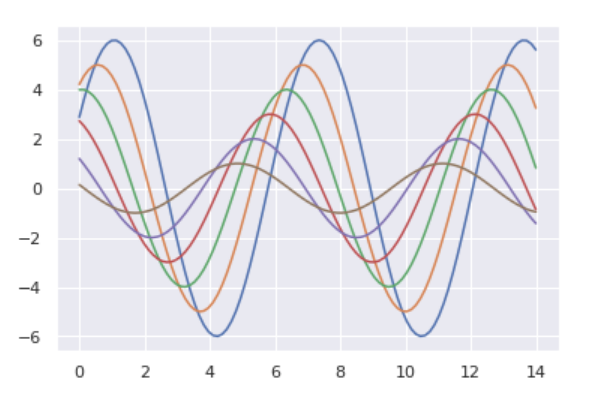``````sns.set_style("whitegrid")
sinplot()
``````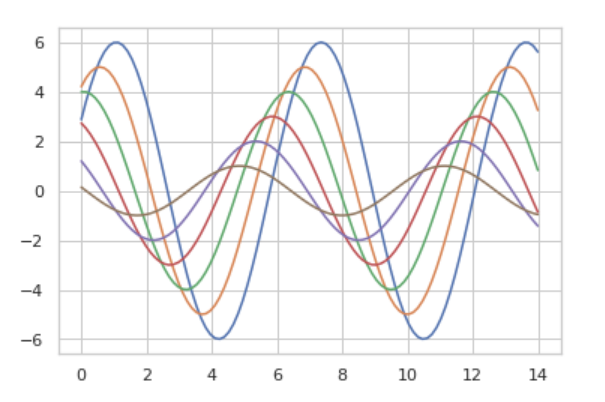``````sns.set_style("dark")
sinplot()
``````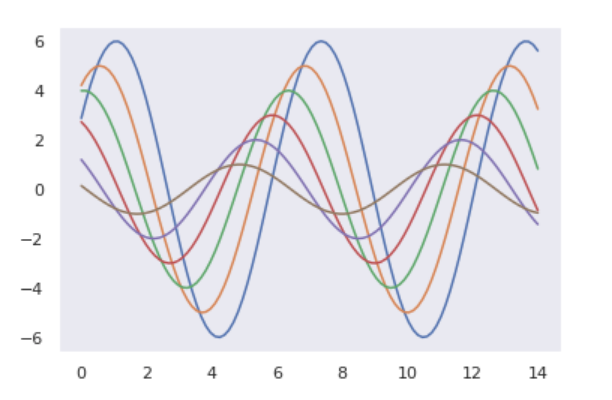``````sns.set_style("white")
sinplot()
``````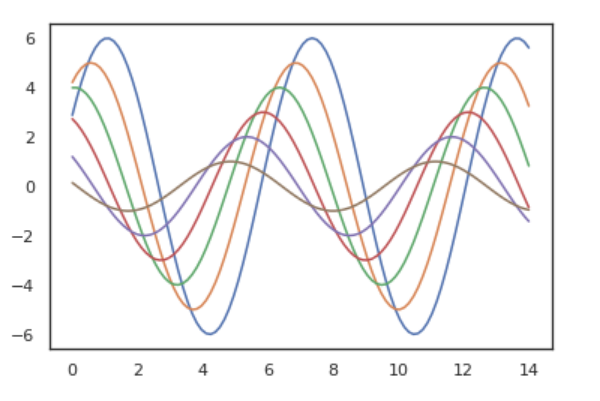context 中可以使用的参数有：

• paper
• talk
• poster
• notebook

### 颜色

#### 色板

color_palette() 允许传入任何 Matplotlib 所支持的颜色，不传入参数时表示使用默认颜色。并且还可以调用 set_palette() 方法来设置所有图的颜色。

``````sns.palplot(sns.color_palette())
````````````sns.palplot(sns.color_palette('hls', 12))
``````• l-亮度 lightness
• s-饱和 saturation

``````data = np.random.normal(size=(20, 12)) + np.arange(12) / 2
sns.boxplot(data=data,palette=sns.color_palette("hls", 12))
``````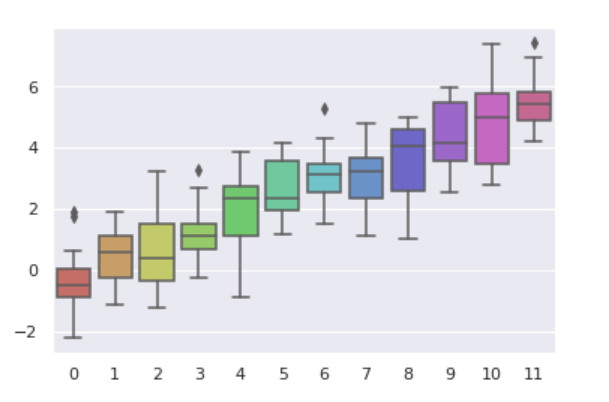#### 连续色板

``````sns.palplot(sns.color_palette("Blues"))
````````````sns.palplot(sns.color_palette('Blues_r'))
``````